# SSAT Upper Level Math : How to find the slope of perpendicular lines

## Example Questions

← Previous 1

### Example Question #61 : Lines

The equation for one line is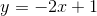. What is the slope of the line that is perpendicular to this line?Explanation:

A line is perpendicular to another if their slopes are negative reciprocals of each other.

Since the slope of the given line is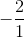, the negative reciprocal would be.

### Example Question #1 : How To Find The Slope Of A Perpendicular Line

What is the slope of any line perpendicular to 2y = 4x +3 ?

½

– 4

2

– ½

– ½

Explanation:

First, we must solve the equation for y to determine the slope:  y = 2x + 3/2

By looking at the coefficient in front of x, we know that the slope of this line has a value of 2. To fine the slope of any line perpendicular to this one, we take the negative reciprocal of it:

slope = m , perpendicular slope = – 1/m

slope = 2 , perpendicular slope = – 1/2

### Example Question #1 : How To Find The Slope Of A Perpendicular Line

What line is perpendicular to 2x + y = 3 at (1,1)?

x + 2y - 3

3x + 2y = 1

2x + 3y = 1

x – 2y = -1

x – 2y = -1

Explanation:

Find the slope of the given line.  The perpendicular slope will be the opposite reciprocal of the original slope.  Use the slope-intercept form (y = mx + b) and substitute in the given point and the new slope to find the intercept, b.  Convert back to standard form of an equation:  ax + by =  c.

### Example Question #1 : How To Find The Slope Of A Perpendicular Line

What is the slope of the line perpendicular to the line given by the equation

6x – 9y +14 = 0

6

-2/3

-1/6

2/3

-3/2

-3/2

Explanation:

First rearrange the equation so that it is in slope-intercept form, resulting in y=2/3 x + 14/9. The slope of this line is 2/3, so the slope of the line perpendicular will have the opposite reciprocal as a slope, which is -3/2.

### Example Question #1 : How To Find The Slope Of A Perpendicular Line

What is the slope of the line perpendicular to the line represented by the equation y = -2x+3?

2/3

-2/3

1/2

2

-1/2

1/2

Explanation:

Perpendicular lines have slopes that are the opposite of the reciprocal of each other. In this case, the slope of the first line is -2. The reciprocal of -2 is -1/2, so the opposite of the reciprocal is therefore 1/2.

### Example Question #1 : How To Find The Slope Of A Perpendicular Line

Find the slope of a line perpendicular to the line y = –3x – 4.

1/3

1/4

4

–3

1/3

Explanation:

First we must find the slope of the given line. The slope of y = –3x – 4 is –3. The slope of the perpendicular line is the negative reciprocal. This means you change the sign of the slope to its opposite: in this case to 3. Then find the reciprocal by switching the denominator and numerator to get 1/3; therefore the slope of the perpendicular line is 1/3.

### Example Question #6 : How To Find The Slope Of A Perpendicular Line

What is the slope of a line perpendicular to the following: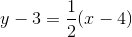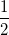Explanation:

The question puts the line in point-slope form y – y1 = m(x  x1), where m is the slope. Therefore, the slope of the original line is 1/2.  A line perpendicular to another has a slope that is the negative reciprocal of the slope of the other line. The negative reciprocal of the original line is 2, and is thus the slope of its perpendicular line.

### Example Question #1 : How To Find The Slope Of A Perpendicular Line

A line is defined by the following equation: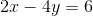What is the slope of a line that is perpendicular to the line above?Explanation:

The equation of a line iswhereis the slope.

Rearrange the equation to match this: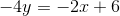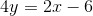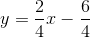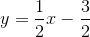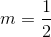For the perpendicular line, the slope is the negative reciprocal;

therefore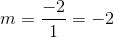### Example Question #71 : Coordinate Geometry

Find the slope of the line perpendicular to the line that has the equation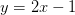.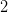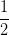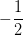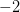Explanation:

Perpendicular lines have slopes that are negative reciprocals of each other. In other words, change the sign and flip the fraction around.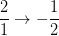### Example Question #72 : Coordinate Geometry

Find the slope of a line perpendicular to the line with the equation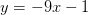.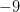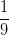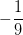Explanation:

Perpendicular lines have slopes that are negative reciprocals of each other. In other words, change the sign and flip the fraction around to find the slope of the line perpendicular to the given one.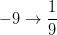← Previous 1

### All SSAT Upper Level Math Resources